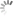## 入门 | 数据科学初学者必知的NumPy基础知识

x

选自TowardsDataScience

作者：Ehi Aigiomawu

机器之心编译

参与：李诗萌、路

本文介绍了一些 NumPy 基础知识，适合数据科学初学者学习掌握。

NumPy（Numerical Python）是 Python 中的一个线性代数库。对每一个数据科学或机器学习 Python 包而言，这都是一个非常重要的库，SciPy（Scientific Python）、Mat-plotlib（plotting library）、Scikit-learn 等都在一定程度上依赖 NumPy。

对数组执行数学运算和逻辑运算时，NumPy 是非常有用的。在用 Python 对 n 维数组和矩阵进行运算时，NumPy 提供了大量有用特征。

这篇教程介绍了数据科学初学者需要了解的 NumPy 基础知识，包括如何创建 NumPy 数组、如何使用 NumPy 中的广播机制、如何获取值以及如何操作数组。更重要的是，大家可以通过本文了解到 NumPy 在 Python 列表中的优势：更简洁、更快速地读写项、更方便、更高效。

本教程将使用 Jupyter notebook 作为编辑器。

让我们开始吧！

安装 NumPy

如果你已经装有 Anaconda，那么你可以使用以下命令通过终端或命令提示符安装 NumPy：

`conda install numpy`

如果你没有 Anaconda，那么你可以使用以下命令从终端上安装 NumPy：

`pip install numpy`

安装好 NumPy 后，你就可以启动 Jupyter notebook 开始学习了。接下来从 NumPy 数组开始。

NumPy 数组

NumPy 数组是包含相同类型值的网格。NumPy 数组有两种形式：向量和矩阵。严格地讲，向量是一维数组，矩阵是多维数组。在某些情况下，矩阵只有一行或一列。

首先将 NumPy 导入 Jupyter notebook：

`import numpy as np`

从 Python 列表中创建 NumPy 数组

我们先创建一个 Python 列表：

`my_list = [1, 2, 3, 4, 5]`

通过这个列表，我们可以简单地创建一个名为 my_numpy_list 的 NumPy 数组，显示结果：

`my_numpy_list = np.array(my_list)my_numpy_list #This line show the result of the array generated`

刚才我们将一个 Python 列表转换成一维数组。要想得到二维数组，我们要创建一个元素为列表的列表，如下所示：

`second_list = [[1,2,3], [5,4,1], [3,6,7]]new_2d_arr = np.array(second_list)new_2d_arr #This line show the result of the array generated`

我们已经成功创建了一个有 3 行 3 列的二维数组。

使用 arange() 内置函数创建 NumPy 数组

与 Python 的 range() 内置函数相似，我们可以用 arange() 创建一个 NumPy 数组。

`my_list = np.arange(10)#ORmy_list = np.arange(0,10)`

这产生了 0~10 的十个数字。

要注意的是 arange() 函数中有三个参数。第三个参数表示步长。例如，要得到 0~10 中的偶数，只需要将步长设置为 2 就可以了，如下所示：

`my_list = np.arange(0,11,2)`

还可以创建有 7 个 0 的一维数组：

`my_zeros = np.zeros(7)`

也可以创建有 5 个 1 的一维数组：

`my_ones = np.ones(5)`

同样，我们可以生成内容都为 0 的 3 行 5 列二维数组：

`two_d = np.zeros((3,5))`

使用 linspace() 内置函数创建 NumPy 数组

linspace() 函数返回的数字都具有指定的间隔。也就是说，如果我们想要 1 到 3 中间隔相等的 15 个点，我们只需使用以下命令：

`lin_arr = np.linspace(1, 3, 15)`

该命令可生成一维向量。

与 arange() 函数不同，linspace() 的第三个参数是要创建的数据点数量。

在 NumPy 中创建一个恒等矩阵

处理线性代数时，恒等矩阵是非常有用的。一般而言，恒等矩阵是一个二维方矩阵，也就是说在这个矩阵中列数与行数相等。有一点要注意的是，恒等矩阵的对角线都是 1，其他的都是 0。恒等矩阵一般只有一个参数，下述命令说明了要如何创建恒等矩阵：

`my_matrx = np.eye(6) #6 is the number of columns/rows you want`

用 NumPy 创建一个随机数组成的数组

我们可以使用 rand()、randn() 或 randint() 函数生成一个随机数组成的数组。

使用 random.rand()，我们可以生成一个从 0~1 均匀产生的随机数组成的数组。

例如，如果想要一个由 4 个对象组成的一维数组，且这 4 个对象均匀分布在 0~1，可以这样做：

`my_rand = np.random.rand(4)`

如果我们想要一个有 5 行 4 列的二维数组，则：

`my_rand = np.random.rand(5, 4)my_rand`

使用 randn()，我们可以从以 0 为中心的标准正态分布或高斯分布中产生随机样本。例如，我们这样生成 7 个随机数：

`my_randn = np.random.randn(7)my_randn`

绘制结果后会得到一个正态分布曲线。

同样地，如需创建一个 3 行 5 列的二维数组，这样做即可：

`np.random.randn(3,5)`

最后，我们可以使用 randint() 函数生成整数数组。randint() 函数最多可以有三个参数：最小值（包含），最大值（不包含）以及数组的大小。

`np.random.randint(20) #generates a random integer exclusive of 20np.random.randint(2, 20) #generates a random integer including 2 but excluding 20np.random.randint(2, 20, 7) #generates 7 random integers including 2 but excluding 20`

将一维数组转换成二维数组

先创建一个有 25 个随机整数的一维数组：

`arr = np.random.rand(25)`

然后使用 reshape() 函数将其转换为二维数组：

`arr.reshape(5,5)`

注意：reshape() 仅可转换成行列数目相等，且行列数相乘后要与元素数量相等。上例中的 arr 包含 25 个元素，因此只能重塑为 5*5 的矩阵。

定位 NumPy 数组中的最大值和最小值

使用 max() 和 min() 函数，我们可以得到数组中的最大值或最小值：

`arr_2 = np.random.randint(0, 20, 10) arr_2.max() #This gives the highest value in the array arr_2.min() #This gives the lowest value in the array`

使用 argmax() 和 argmin() 函数，我们可以定位数组中最大值和最小值的索引：

`arr_2.argmax() #This shows the index of the highest value in the array arr_2.argmin() #This shows the index of the lowest value in the array`

假设存在大量数组，而你需要弄清楚数组的形态，你想知道这个数组是一维数组还是二维数组，只需要使用 shape 函数即可：

`arr.shape`

从 NumPy 数组中索引／选择多个元素（组）

在 NumPy 数组中进行索引与 Python 类似，只需输入想要的索引即可：

`my_array = np.arange(0,11)my_array #This gives us the value of element at index 8`

为了获得数组中的一系列值，我们可以使用切片符「:」，就像在 Python 中一样：

`my_array[2:6] #This returns everything from index 2 to 6(exclusive)my_array[:6] #This returns everything from index 0 to 6(exclusive)my_array[5:] #This returns everything from index 5 to the end of the array.`

类似地，我们也可以通过使用 [ ][ ] 或 [,] 在二维数组中选择元素。

使用 [ ][ ] 从下面的二维数组中抓取出值「60」：

`two_d_arr = np.array([[10,20,30], [40,50,60], [70,80,90]])two_d_arr #The value 60 appears is in row index 1, and column index 2`

使用 [,] 从上面的二维数组中抓取出值「20」：

`two_d_arr[0,1]`

也可以用切片符抓取二维数组的子部分。使用下面的操作从数组中抓取一些元素：

`two_d_arr[:1, :2] # This returns [[10, 20]]two_d_arr[:2, 1:] # This returns ([[20, 30], [50, 60]])two_d_arr[:2, :2] #This returns ([[10, 20], [40, 50]])`

我们还可以索引一整行或一整列。只需使用索引数字即可抓取任意一行：

`two_d_arr #This grabs row 0 of the array ([10, 20, 30])two_d_arr[:2] #This grabs everything before row 2 ([[10, 20, 30], [40, 50, 60]])`

还可以使用 &、|、<、> 和 == 运算符对数组执行条件选择和逻辑选择，从而对比数组中的值和给定值：

`new_arr = np.arange(5,15)new_arr > 10 #This returns TRUE where the elements are greater than 10 [False, False, False, False, False, False, True, True, True, True]`

现在我们可以输出符合上述条件的元素：

`bool_arr = new_arr > 10new_arr[bool_arr] #This returns elements greater than 10 [11, 12, 13, 14]new_arr[new_arr>10] #A shorter way to do what we have just done`

组合使用条件运算符和逻辑运算符，我们可以得到值大于 6 小于 10 的元素：

`new_arr[(new_arr>6) & (new_arr<10)]`

预期结果为：([7, 8, 9])

广播机制

广播机制是一种快速改变 NumPy 数组中的值的方式。

`my_array[0:3] = 50#Result is: [50, 50, 50, 3, 4, 5, 6, 7, 8, 9, 10]`

在这个例子中，我们将索引为 0 到 3 的元素的初始值改为 50。

对 NumPy 数组执行数学运算

`arr = np.arange(1,11)arr * arr #Multiplies each element by itself arr - arr #Subtracts each element from itselfarr + arr #Adds each element to itselfarr / arr #Divides each element by itself`

我们还可以对数组执行标量运算，NumPy 通过广播机制使其成为可能：

`arr + 50 #This adds 50 to every element in that array`

NumPy 还允许在数组上执行通用函数，如平方根函数、指数函数和三角函数等。

`np.sqrt(arr) #Returns the square root of each element np.exp(arr) #Returns the exponentials of each elementnp.sin(arr) #Returns the sin of each elementnp.cos(arr) #Returns the cosine of each elementnp.log(arr) #Returns the logarithm of each elementnp.sum(arr) #Returns the sum total of elements in the arraynp.std(arr) #Returns the standard deviation of in the array`

我们还可以在二维数组中抓取行或列的总和：

`mat = np.arange(1,26).reshape(5,5)mat.sum() #Returns the sum of all the values in matmat.sum(axis=0) #Returns the sum of all the columns in matmat.sum(axis=1) #Returns the sum of all the rows in mat`

现在，这篇 NumPy 教程进入了尾声！希望对大家有所帮助。本文为机器之心编译，转载请联系本公众号获得授权

------------------------------------------------

加入机器之心（全职记者/实习生）：hr@jiqizhixin.com

投稿或寻求报道：editor@jiqizhixin.com

广告&商务合作：bd@jiqizhixin.com

© 1997-2019 网易公司版权所有 About NetEase | 公司简介 | 联系方法 | 招聘信息 | 客户服务 | 隐私政策 | 广告服务 | 网站地图 | 意见反馈 | 不良信息举报

#### 机器之心#### 机器之心

3691

89834列表加载中...

x